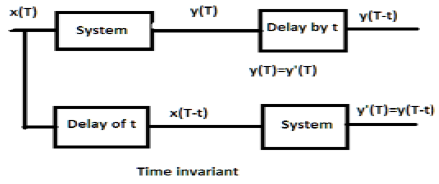Courses

Time Invariant & Time Variant Systems Notes | Study Digital Signal Processing - Electrical Engineering (EE)

Electrical Engineering (EE): Time Invariant & Time Variant Systems Notes | Study Digital Signal Processing - Electrical Engineering (EE)

The document Time Invariant & Time Variant Systems Notes | Study Digital Signal Processing - Electrical Engineering (EE) is a part of the Electrical Engineering (EE) Course Digital Signal Processing.
All you need of Electrical Engineering (EE) at this link: Electrical Engineering (EE)

For a time-invariant system, the output and input should be delayed by some time unit. Any delay provided in the input must be reflected in the output for a time invariant system.Examples

a) y(T) = x(2T)

If the above expression, it is first passed through the system and then through the time delay (as shown in the upper part of the figure); then the output will become x(2T−2t). Now, the same expression is passed through a time delay first and then through the system (as shown in the lower part of the figure). The output will become x(2T−t)x.

Hence, the system is not a time-invariant system.

b) y(T) = sin[x(T)]

If the signal is first passed through the system and then through the time delay process, the output be sinx(T−t). Similarly, if the system is passed through the time delay first then through the system then output will be sinx(T−t). We can see clearly that both the outputs are same. Hence, the system is time invariant.

For a time variant system, also, output and input should be delayed by some time constant but the delay at the input should not reflect at the output. All time scaling cases are examples of time variant system. Similarly, when coefficient in the system relationship is a function of time, then also, the system is time variant.Examples

a) y(t) = x[cosT]

If the above signal is first passed through the system and then through the time delay, the output will be xcos(T−t). If it is passed through the time delay first and then through the system, it will be x(cosT−t). As the outputs are not same, the system is time variant.

b) y(T) = cosT.x(T)

If the above expression is first passed through the system and then through the time delay, then the output will be cos(T−t)x(T−t). However, if the expression is passed through the time delay first and then through the system, the output will be cosT.x(T−t). As the outputs are not same, clearly the system is time variant.

The document Time Invariant & Time Variant Systems Notes | Study Digital Signal Processing - Electrical Engineering (EE) is a part of the Electrical Engineering (EE) Course Digital Signal Processing.
All you need of Electrical Engineering (EE) at this link: Electrical Engineering (EE)Use Code STAYHOME200 and get INR 200 additional OFF Use Coupon Code

Top Courses for Electrical Engineering (EE)Digital Signal Processing

3 videos|50 docs|54 tests

Top Courses for Electrical Engineering (EE)Track your progress, build streaks, highlight & save important lessons and more!

,

,

,

,

,

,

,

,

,

,

,

,

,

,

,

,

,

,

,

,

,

;